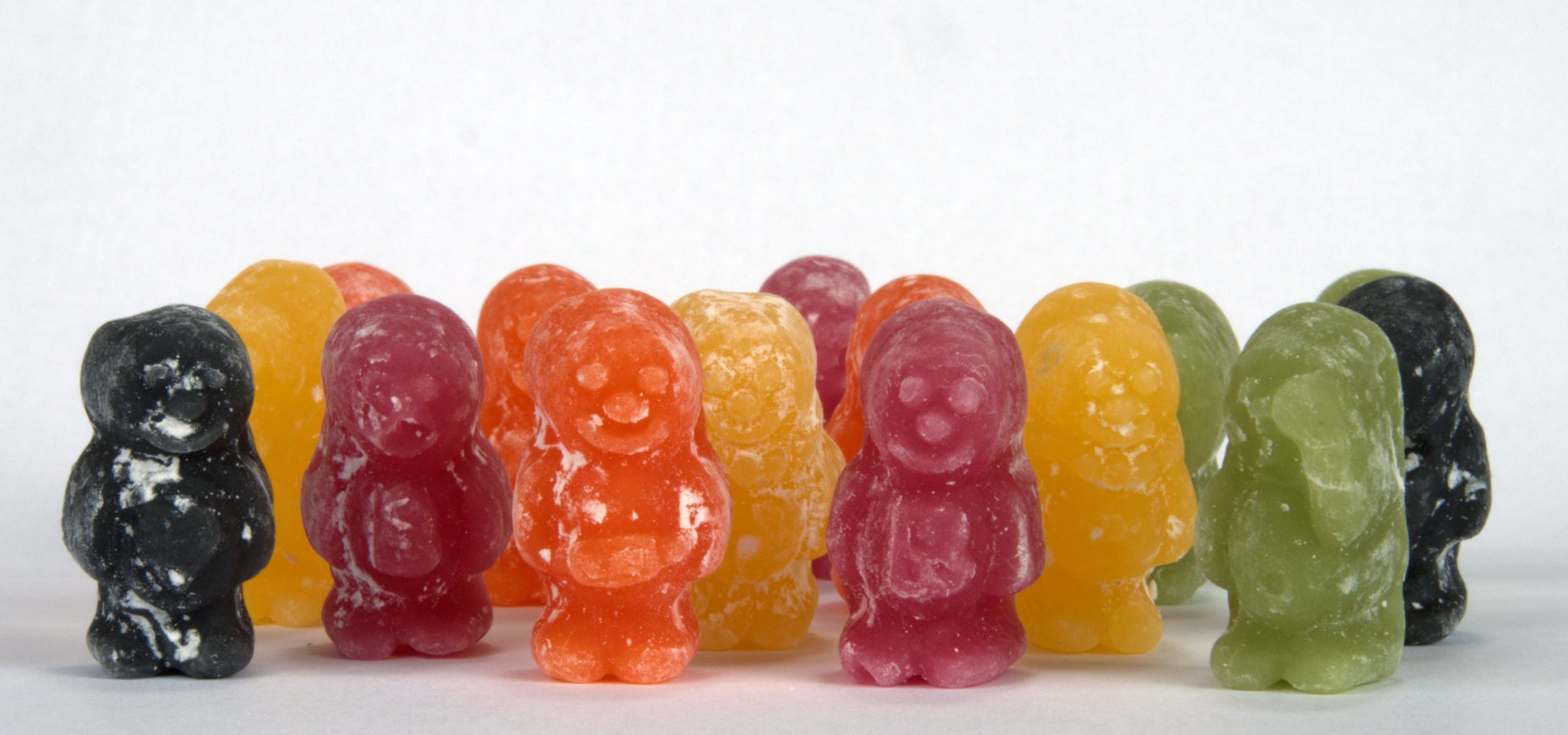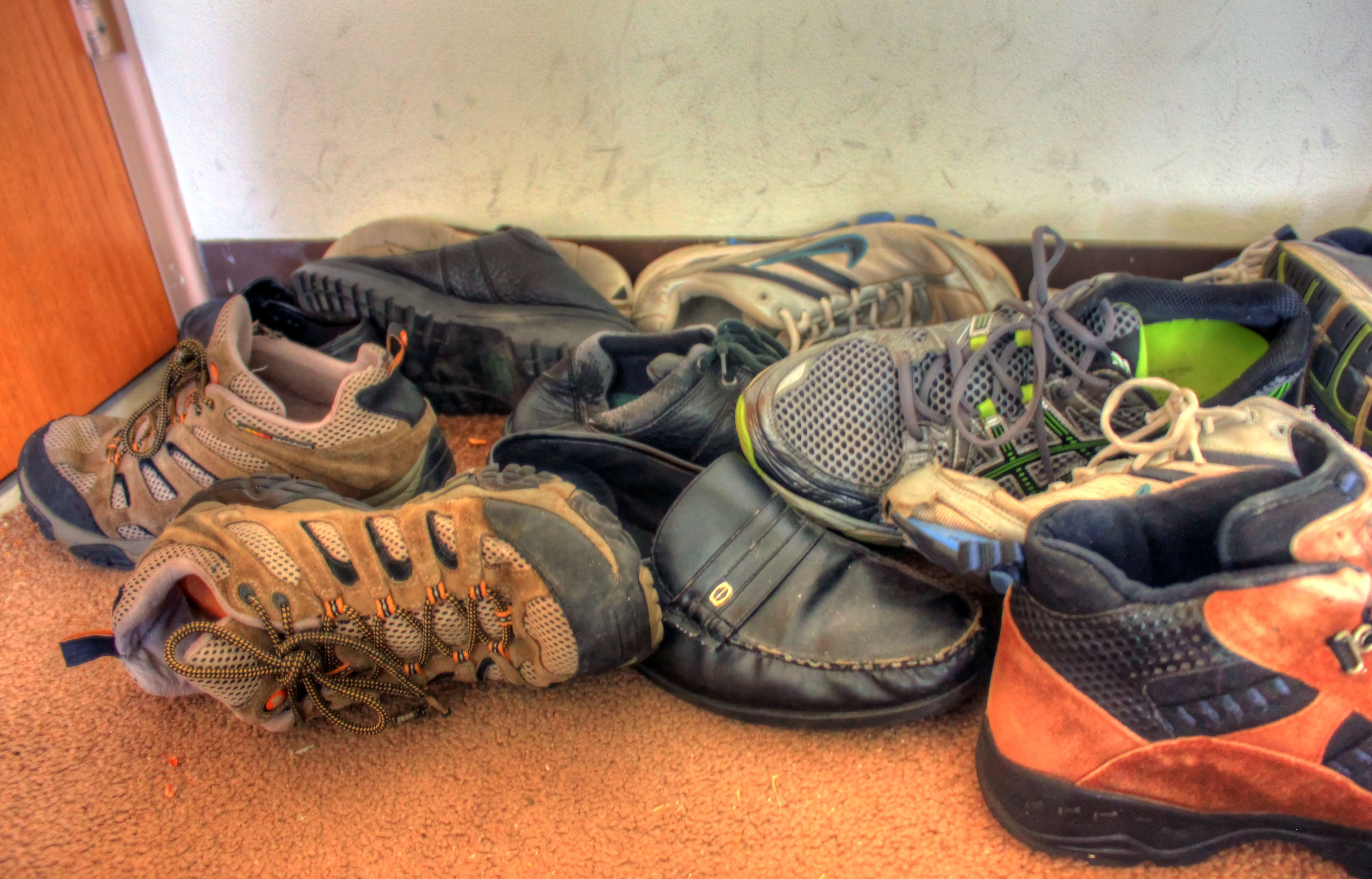# Discrete Data and Continuous Data

There are two main types of data: qualitative data (data that describes something) and quantitative data (data that tells you how many, how long, how high or how wide). Quantitative data is numerical data as it involves numbers. In this article we're interested in the different types of quantitative (or numerical) data.

Quantitative data can be discrete or continuous. In their simplest forms,

• Discrete data is data that you count.
• Continuous data is data that you measure.

## Discrete Data

It is a bit of an oversimplification to say that discrete data is data that you count. Of course data that you count is discrete, but, more precisely, discrete data is data that is only allowed to have certain values. If you think of data being placed along a number line, discrete data is the data for which only certain points on the number line make sense as data values. It won't fill in the whole number line. There will be gaps!

For example, if you were recording the number of pets that each person in your class has at home, only whole numbers of pets would make sense. You can't have half a pet, and you can't have a negative pet. So, the number of pets that each person in your class has is an example of discrete data.

Another example would be to record shoe sizes. Shoe sizes like $5$ and $7 \;\dfrac{1}{2}$ make sense. Shoe sizes like $6.3654$ do not. So, shoe sizes are an example of discrete data.

Let's look at a few more examples of discrete data.The number of jelly babies in each $200 \text{g}$ packet of jelly babies is an example of discrete data. Packets contain only whole jelly babies, not half jelly babies. However, the weights of the jelly babies in a packet would be an example of continuous data (see later).

The number of people sitting in the members stand at the SCG on each day of the summer is also an example of discrete data. There can only be whole people. Again, the weights or heights of the people would be an example of continuous data.The number of petals on each of the flowers in a garden bed is an example of discrete data. The lengths of the petals would be an example of continuous data.

The number of pairs of shoes each person in your class owns is an example of discrete data. You can have half pairs of shoes, but they should probably be thrown out. However, quarter pairs of shoes don't make a lot of sense, unless the dog's been having a chew. They should definitely be thrown out! The sizes of the shoes worn by people in your class is also an example of discrete data as we discussed earlier.## Continuous Data

Continuous Data is data that you can measure somehow. For example, it might be a collection of heights, times or weights. If you place continuous data on a number line, it can be plotted at any value. It fills in the whole number line. There might be a minimum and a maximum possible value, but it can take any value in between these.

We've already mentioned some examples of continuous data. Let's have a look at a few more.The heights of gum trees in a patch of bushland form an example of continuous data. Trees can have any height between some minimum and maximum values. The number of trees in different patches of bushland would be an example of discrete data.

The lengths of fern fronds in a fernery would be an example of continuous data. Fronds can take any length between some minimum and maximum values. The number of fern fronds on each plant would be an example of discrete data.The weights of ducklings form an example of continuous data. Ducklings can take on any weight between some minimum and maximum possible weights. The number of ducklings in each brood on a lake is an example of discrete data.

The times people take to complete a $100$ metre freestyle race form an example of continuous data. Times can be measured to any fraction of a second.## Conclusion

There are many more examples of discrete and continuous data. Why don't you see if you can think of some different data sets and decide whether they represent discrete or continuous data? Remember, discrete data can only take on certain values. Continuous data can take on any values within a given range.

### Description

This chapter series is on Data and is suitable for Year 10 or higher students, topics include

• Accuracy and Precision
• Calculating Means From Frequency Tables
• Correlation
• Cumulative Tables and Graphs
• Discrete and Continuous Data
• Finding the Mean
• Finding the Median
• FindingtheMode
• Formulas for Standard Deviation
• Grouped Frequency Distribution
• Normal Distribution
• Outliers
• Quartiles
• Quincunx
• Quincunx Explained
• Range (Statistics)
• Skewed Data
• Standard Deviation and Variance
• Standard Normal Table
• Univariate and Bivariate Data
• What is Data

### Audience

Year 10 or higher students, some chapters suitable for students in Year 8 or higher

### Learning Objectives

Learn about topics related to "Data"

Author: Subject Coach
You must be logged in as Student to ask a Question.